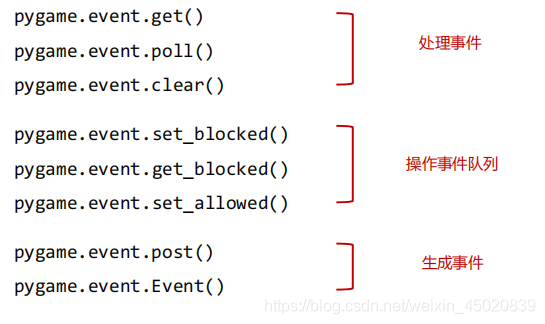﻿ pygame 键盘事件的实践_python_脚本之家
pythonpygame 键盘事件的实践

Pygame事件

pygame.event.EventType
'''
• 事件本质上是一种封装后的数据类型（对象）
• EventType是Pygame的一个类，表示事件类型
• 事件类型只有属性，没有方法
• 用户可自定义新的事件类型
'''

事件类型及属性事件处理函数键盘事件及类型的使用

键盘事件及属性

pygame.event.KEYDOWN  #键盘按下事件
pygame.event.KEYUP   #键盘释放事件

event.unicode   #按键的unicode码，平台有关，不推荐使用
event.key   # 按键的常量名称
event.mod   # 按键修饰符的组合值

K_BACKSPACE 退格键（Backspace）
K_TAB 制表键（Tab）
K_CLEAR 清除键
K_RETURN 回车键（Enter）
K_PAUSE 暂停键 （Pause）
K_ESCAPE 退出键（Escape）
K_SPACE 空格键 （Space）
K_EXCLAIM 感叹号
K_QUOTEDBL 双引号
K_HASH 井号
K_DOLLAR 美元符号
K_AMPERSAND and 符号
K_QUOTE 单引号
K_LEFTPAREN 左小括号
K_RIGHTPAREN 右小括号
K_ASTERISK 星号
K_PLUS 加号
K_COMMA 逗号
K_MINUS 减号
K_PERIOD 句号
K_SLASH 正斜杠
K_0 0
K_1 1
K_2 2
K_3 3
K_4 4
K_5 5
K_6 6
K_7 7
K_8 8
K_9 9
K_COLON 冒号
K_SEMICOLON 分号
K_LESS 小于号
K_EQUALS 等于号
K_GREATER 大于号
K_QUESTION 问号
K_AT @ 符号
K_LEFTBRACKET 左中括号
K_BACKSLASH 反斜杠
K_RIGHTBRACKET 右中括号
K_CARET 脱字符
K_UNDERSCORE 下划线
K_BACKQUOTE 重音符
K_a a
K_b b
K_c c
K_d d
K_e e
K_f f
K_g g
K_h h
K_i i
K_j j
K_k k
K_l l
K_m m
K_n n
K_o o
K_p p
K_q q
K_r r
K_s s
K_t t
K_u u
K_v v
K_w w
K_x x
K_y y
K_z z
K_DELETE 删除键（delete）
K_KP0 0（小键盘）
K_KP1 1（小键盘）
K_KP2 2 （小键盘）
K_KP3 3（小键盘）
K_KP4 4（小键盘）
K_KP5 5 （小键盘）
K_KP6 6 （小键盘）
K_KP7 7 （小键盘）
K_KP8 8 （小键盘）
K_KP9 9 （小键盘）
K_KP_PERIOD 句号（小键盘）
K_KP_DIVIDE 除号（小键盘）
K_KP_MULTIPLY 乘号（小键盘）
K_KP_MINUS 减号（小键盘）
K_KP_PLUS 加号（小键盘）
K_KP_ENTER 回车键（小键盘）
K_KP_EQUALS 等于号（小键盘）
K_UP 向上箭头（up arrow）
K_DOWN 向下箭头（down arrow）
K_RIGHT 向右箭头（right arrow）
K_LEFT 向左箭头（left arrow）
K_INSERT 插入符（insert）
K_HOME Home 键（home）
K_END End 键（end）
K_PAGEUP 上一页（page up）
K_PAGEDOWN 下一页（page down）
K_F1 F1
K_F2 F2
K_F3 F3
K_F4 F4
K_F5 F5
K_F6 F6
K_F7 F7
K_F8 F8
K_F9 F9
K_F10 F10
K_F11 F11
K_F12 F12
K_F13 F13
K_F14 F14
K_F15 F15
K_NUMLOCK 数字键盘锁定键
K_CAPSLOCK 大写字母锁定键
K_SCROLLOCK 滚动锁定键
K_RSHIFT 右边的 shift 键
K_LSHIFT 左边的 shift 键
K_RCTRL 右边的 ctrl 键
K_LCTRL 左边的 ctrl 键
K_RALT 右边的 alt 键
K_LALT 左边的 alt 键
K_RMETA 右边的元键
K_LMETA 左边的元键
K_LSUPER 左边的 Window 键
K_RSUPER 右边的 Window 键
K_MODE 模式转换键
K_HELP 帮助键
K_PRINT 打印屏幕键
K_SYSREQ 魔术键
K_BREAK 中断键
K_POWER 电源键
K_EURO 欧元符号

按键的修饰符

KMOD_NONE 没同时按下组合键
KMOD_LSHIFT 同时按下左边的 shift 键
KMOD_RSHIFT 同时按下右边的 shift 键
KMOD_SHIFT 同时按下 shift 键
KMOD_CAPS 同时按下大写字母锁定键
KMOD_LCTRL 同时按下左边的 ctrl 键
KMOD_RCTRL 同时按下右边的 ctrl 键
KMOD_CTRL 同时按下 ctrl 键
KMOD_LALT 同时按下左边的 alt 键
KMOD_RALT 同时按下右边的 alt 键
KMOD_ALT 同时按下 alt 键
KMOD_LMETA 同时按下左边的元键
KMOD_RMETA 同时按下右边的元键
KMOD_META 同时按下元键
KMOD_NUM 同时按下数字键盘锁定键
KMOD_MODE 同时按下模式转换键

event.mod
'''

event.mod = KMOD_ALT | KMOD_SHIFT
'''

import pygame,sys

pygame.init()

s = pygame.display.set_mode((600,400))
pygame.display.set_caption("hi 键盘")
while True:
for event in pygame.event.get():
if event.type == pygame.QUIT:
sys.exit()
elif event.type == pygame.KEYDOWN:
if event.unicode == "":
print("#",event.key,event.mod)
else:
print(event.unicode,event.key,event.mod)
pygame.display.update()